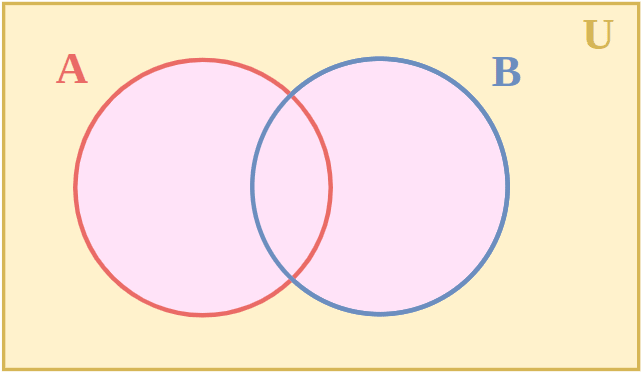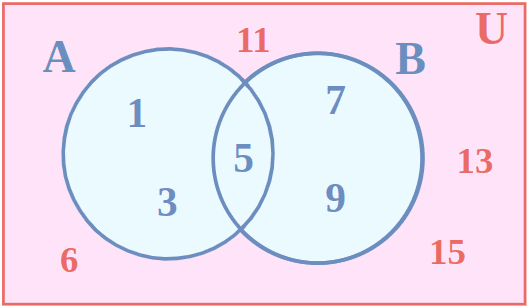# Universal Sets

Universal Set is a set that has all the elements associated with a given set, without any repetition. Suppose we have two sets P = {1, 3, 5} and Q = {2, 4, 6} then the universal set of P and Q is U = {1, 2, 3, 4, 5, 6}. We generally use U to denote universal sets.

Universal Set is a type of set that is used to represent all the possible elements associated with a set. It is widely used in probability to represent all possible sample space of an event. In this article, we will learn about, Universal sets, Examples of Universal Sets, Complements of Universal Sets, Venn Diagram of Universal Sets, and others in detail.

## Universal Set Definition

Universal Set is the set of all the sets, i.e., it contains all the elements present in all the sets given. The universal set is represented as U, and it is represented as a rectangle in the Venn diagram, all the other sets are drawn inside the rectangle, this is done to show that the universal set contains all the possible elements of all the sets.

Assume set A and set B,

• Set A = {1, 2, 3, 4}
• Set B= {2, 4, 5, 6, 7, 8}

Then the universal set containing set A and set B is, U and is defined as,

U= {1, 2, 3, 4, 5, 6, 7, 8}

We also define that set of complex number is the universal set of the number because it contains all the numbers including, Imaginary Numbers, Real Numbers, Rational Numbers, Irrational Numbers, Integers, Whole Numbers, Natural Numbers, etc.

### Universal Sets Symbol

We use English Letter ‘U’ for representing Universal Set. It contain all the elements associate with the concerning sets.

### Universal Sets Examples

Examples of universal set are,

• Set of Complex Number is the universal set of all the numbers as it contains all the numbers.
• Suppose we have three sets,
• set A = (Alphabets in Government)
• set B = {a, e, i, o, u}
• set C = (Alphabets in Country)

Then the universal set of set A, set B, and set C is,

U = (All alphabets in English Language)

## Complements of Universal Sets

We know that universal set U is a set that contains all the elements of subsets, so compelement of the universal set is a set that contain no elements of the subsets. Thus, we can say that, the complement of the universal set is an Empty Set Or Null Set. A null set is denoted by ‘{}’ or ‘Φ’ symbol.

## Venn Diagram of Universal Set

Venn Diagrams are used to represent relation between sets using pictorials methods. We use circles to represent various sets. A universal set is a set that contains all the elements associated with all the sets in considerations. We use rectangles to represents Universal Sets.

The image added below shows the universal set U which contains three sets A, B and C. The region of intersection of sets is also shown.## Difference Between Universal Set and Union of Sets

Universal set is a set that contain all the elements associated with the subset in concern (it can also have more elements that are not prsesnt in the subsets), where as union of two sets only contain the elements that are present in the two sets any extra elements is not allowed in the union of to sets.

The basic differences between Universal Set and Union of Sets can be understood with the table added below:

Universal Set

Union of Sets

A set containing all the elements associated with the concerning set is called the universal set.

A set containing elements that are present in the sets whose union is to be taken is called the union of set.

Universal set is denoted using letter “U”

Union of two sets is an operation and is denoted using union operator ‘∪’. Suppose we have to find union of set A and set B then, it is represented as,

A∪B and is read as A union B

Universal set can contain some extra elements other than the elements in the subset.

Union of a set can not have more than elements that are in the subset.

Example,

• set A = {1, 3, 5, 7}
• set B = {2, 4, 6, 8}

Universal Set ‘U’

• U = {0, 1, 2, 3, 4, 5, 6, 7, 8, 9}

Example,

• set A = {1, 3, 5, 7}
• set B = {2, 4, 6, 8}

Union of set A and set B

• A∪B = { 1, 2, 3, 4, 5, 6, 7, 8}

## Solved Examples on Universal Sets

Problem 1: Represent the information given below in Venn diagram,

• Set A = {1, 3, 5}
• Set B = {5, 7, 9}

U = {1, 3, 5, 6, 7, 9,11, 13, 15}

Solution:

Given set,

• Set A = {1, 3, 5}
• Set B= {5, 7, 9}

U = {1, 3, 5, 6, 7, 9,11, 13, 15}

Universal set “U” is represented as,Problem 2: Find the universal set of

• set A = {3, 6, 9, 12}
• set B = {1, 2, 3, 4, 5}

Solution:

Given set,

• Set A = {1, 3, 5}
• Set B= {5, 7, 9}

Universal set ‘U’

U = {1, 2, 3, 4, 5, 6, 9, 12}

## FAQs on Universal Sets

### 1. What is a Set?

A set is a well defined collection of distinct objects. It is a concept in mathematics that is used to represent various various group of things. For example suposse in a class a group of students having 150 cm height is a set.

### 2. What is a Universal Set?

Universal set is a set that contains all the elements of the sets associated with the given set. A universal set contains all the elements of the sets.

### 3. What is Union of a Set?

Union of a set is a set that contain all the elements of the sets that union has to be calculated. Suppose we have a set A = {1, 2, 3, 4} and set B = {3, 4, 5, 6} than the union of A and B is A ∪ B and its value is, A ∪ B = {1, 2, 3, 4, 5, 6}

### 4. What is Superset?

A set that contains all the elements of the other set is called the superset of the first set. Suppose we have a set A and set B and if all the elements of set A are contained in set B then set B is called the superset of set A and is represented as, A ⊇ B.

### 5. How is Universal Set Represented?

A universal set is represented using the English alphabet ‘U’.

Whether you're preparing for your first job interview or aiming to upskill in this ever-evolving tech landscape, GeeksforGeeks Courses are your key to success. We provide top-quality content at affordable prices, all geared towards accelerating your growth in a time-bound manner. Join the millions we've already empowered, and we're here to do the same for you. Don't miss out - check it out now!

Previous
Next